# Trees

## THE CONCEPT OF TREES

### Introduction

Trees are used to impose a hierarchical structure on a collection of data items. For example, we need to impose a hierarchical structure on a collection of data items while preparing organizational charts and geneologies to represent the syntactic structure of a source program in compilers. So the study of trees as one of the data structures is important.

Definition of a Tree

A tree is a set of one or more nodes T such that:

1. there is a specially designated node called a root
2. The remaining nodes are partitioned into n disjointed set of nodes T1, T2,…,Tn, each of which is a tree.

A tree strucutre is shown in Figure 21.1.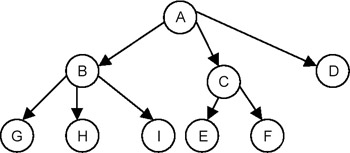Figure 21.1: A tree structure.

This is a tree because it is a set of nodes {A,B,C,D,E,F,G,H,I}, with node A as a root node and the remaining nodes partitioned into three disjointed sets {B,G,H,I}, { C,E,F} and {D}, respectively. Each of these sets is a tree because each satisfies the aforementioned definition properly.

Shown in Figure 21.2 is a structure that is not a tree.Figure 21.2: A non-tree structure.

Even though this is a set of nodes {A,B,C,D,E,F,G,H,I}, with node A as a root node, this is not a tree because the fact that node E is shared makes it impossible to partition nodes B through I into disjointed sets.

Degree of a Node of a Tree

The degree of a node of a tree is the number of subtrees having this node as a root. In other words, the degree is the number of descendants of a node. If the degree is zero, it is called a terminal or leaf node of a tree.

Degree of a Tree

The degree of a tree is defined as the maximum of degree of the nodes of the tree, that is, degree of tree = max (degree(node i) for I = 1 to n)

Level of a Node

We define the level of the node by taking the level of the root node as 1, and incrementing it by 1 as we move from the root towards the subtrees. So the level of all the descendants of the root nodes will be 2. The level of their descendants will be 3, and so on. We then define the depth of the tree to be the maximum value of the level of the node of the tree.

## BINARY TREE AND ITS REPRESENTATION

### Introduction

A binary tree is a special case of tree as defined in the preceding section, in which no node of a tree can have a degree of more than 2. Therefore, a binary tree is a set of zero or more nodes T such that:

1. there is a specially designated node called the root of the tree
2. the remaining nodes are partitioned into two disjointed sets, T1 and T2, each of which is a binary tree. T1 is called the left subtree and T2 is called right subtree, or vice-versa.

A binary tree is shown in Figure 21.3.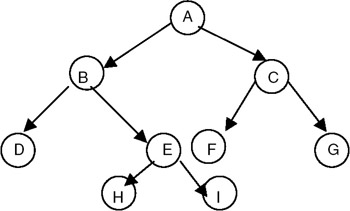Figure 21.3: Binary tree structure.

So, for a binary tree we find that:

1. The maximum number of nodes at level i will be 2i−1
2. If k is the depth of the tree then the maximum number of nodes that the tree can have is

2k − 1 = 2k−1 + 2k−2 + … + 20

Also, there are skewed binary trees, such as the one shown in Figure 21.4.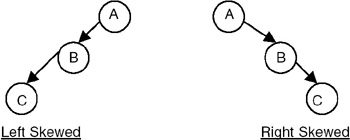Figure 21.4: Skewed trees.

A full binary tree is a binary of depth k having 2k − 1 nodes. If it has < 2k − 1, it is not a full binary tree. For example, for k = 3, the number of nodes = 2k − 1 = 23 − 1 = 8 − 1 = 7. A full binary tree with depth k = 3 is shown in Figure 21.5.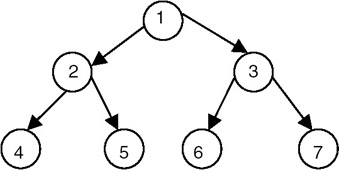Figure 21.5: A full binary tree.

We use numbers from 1 to 2k − 1 as labels of the nodes of the tree.

If a binary tree is full, then we can number its nodes sequentially from 1 to 2k−1, starting from the root node, and at every level numbering the nodes from left to right.

A complete binary tree of depth k is a tree with n nodes in which these n nodes can be numbered sequentially from 1 to n, as if it would have been the first n nodes in a full binary tree of depth k.

A complete binary tree with depth k = 3 is shown in Figure 21.6.Figure 21.6: A complete binary tree.

Representation of a Binary Tree

If a binary tree is a complete binary tree, it can be represented using an array capable of holding n elements where n is the number of nodes in a complete binary tree. If the tree is an array of n elements, we can store the data values of the ith node of a complete binary tree with n nodes at an index i in an array tree. That means we can map node i to the ith index in the array, and the parent of node i will get mapped at an index i/2, whereas the left child of node i gets mapped at an index 2i and the right child gets mapped at an index 2i + 1. For example, a complete binary tree with depth k = 3, having the number of nodes n = 5, can be represented using an array of 5 as shown in Figure 21.7.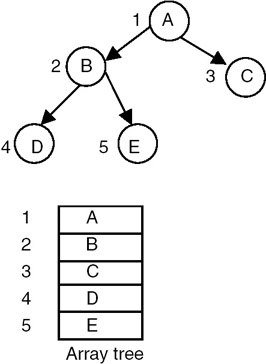Figure 21.7: An array representation of a complete binary tree having 5 nodes and depth 3.

Shown in Figure 21.8 is another example of an array representation of a complete binary tree with depth k = 3, with the number of nodes n = 4.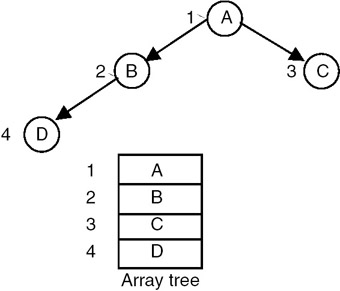Figure 21.8: An array representation of a complete binary tree with 4 nodes and depth 3.

In general, any binary tree can be represented using an array. We see that an array representation of a complete binary tree does not lead to the waste of any storage. But if you want to represent a binary tree that is not a complete binary tree using an array representation, then it leads to the waste of storage as shown in Figure 21.9.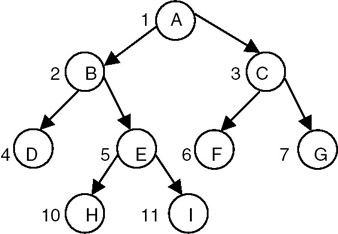Figure 21.9: An array representation of a binary tree.

An array representation of a binary tree is not suitable for frequent insertions and deletions, even though no storage is wasted if the binary tree is a complete binary tree. It makes insertion and deletion in a tree costly. Therefore, instead of using an array representation, we can use a linked representation, in which every node is represented as a structure with three fields: one for holding data, one for linking it with the left subtree, and the third for linking it with right subtree as shown here:

 leftchild data rightchild

We can create such a structure using the following C declaration:

```struct tnode
{
int data
struct tnode *lchild,*rchild;
};
```

A tree representation that uses this node structure is shown in Figure 21.10.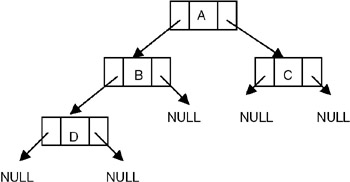Figure 21.10: Linked representation of a binary tree.

## BINARY TREE TRAVERSAL

### Introduction

Order of Traversal of Binary Tree

The following are the possible orders in which a binary tree can be traversed:

 LDR LRD DLR RDL RLD DRL

where L stands for traversing the left subtree, R stands for traversing the right subtree, and D stands for processing the data of the node. Therefore, the order LDR is the order of traversal in which we start with the root node, visit the left subtree, process the data of the root node, and then visit the right subtree. Since the left and right subtrees are also the binary trees, the same procedure is used recursively while visiting the left and right subtrees.

The order LDR is called as inorder; the order LRD is called as postorder; and the order DLR is called as preorder. The remaining three orders are not used. If the processing that we do with the data in the node of tree during the traversal is simply printing the data value, then the output generated for a tree is given in Figure 21.11, using inorder, preorder and postorder as shown.Figure 21.11: A binary tree along with its inorder, preorder and postorder.

If an expression is represented as a binary tree, the inorder traversal of the tree gives us an infix expression, whereas the postorder traversal gives us a postfix expression as shown in Figure 21.12.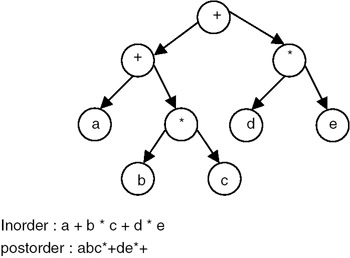Figure 21.12: A binary tree of an expression along with its inorder and postorder.

Given an order of traversal of a tree, it is possible to construct a tree; for example, consider the folowing order:

Inorder = DBEAC

We can construct the binary trees shown in Figure 21.13 by using this order of traversal: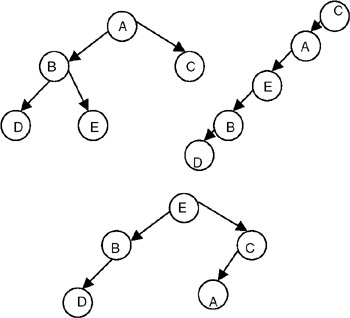Figure 21.13: Binary trees constructed using the given inorder.

Therefore, we conclude that given only one order of traversal of a tree, it is possible to construct a number of binary trees; a unique binary tree cannot be constructed with only one order of traversal. For construction of a unique binary tree, we require two orders, in which one has to be inorder; the other can be preorder or postorder. For example, consider the following orders:

Inorder = DBEAC

Postorder = DEBCA

We can construct the unique binary tree shown in Figure 21.14 by using these orders of traversal: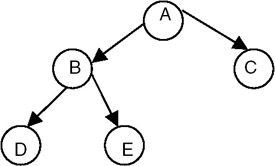Figure 21.14: A unique binary tree constructed using its inorder and postorder.

## BINARY SEARCH TREE

### Introduction

A binary search tree is a binary tree that may be empty, and every node must contain an identifier. An identifier of any node in the left subtree is less than the identifier of the root. An identifier of any node in the right subtree is greater than the identifier of the root. Both the left subtree and right subtree are binary search trees.

A binary search tree is shown in Figure 21.15.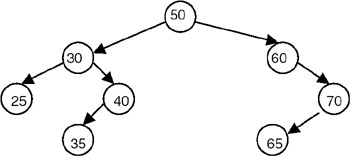Figure 21.15: The binary search tree.

The binary search tree is basically a binary tree, and therefore it can be traversed in inorder, preorder, and postorder. If we traverse a binary search tree in inorder and print the identifiers contained in the nodes of the tree, we get a sorted list of identifiers in ascending order.

A binary search tree is an important search structure. For example, consider the problem of searching a list. If a list is ordered, searching becomes faster if we use a contiguous list and perform a binary search. But if we need to make changes in the list, such as inserting new entries and deleting old entries, using a contiguous list would be much slower, because insertion and deletion in a contiguous list requires moving many of the entries every time. So we may think of using a linked list because it permits insertions and deletions to be carried out by adjusting only a few pointers. But in an n-linked list, there is no way to move through the list other than one node at a time, permitting only sequential access. Binary trees provide an excellent solution to this problem. By making the entries of an ordered list into the nodes of a binary search tree, we find that we can search for a key in O(n logn) steps.

Program: Creating a Binary Search Tree

We assume that every node of a binary search tree is capable of holding an integer data item and that the links can be made to point to the root of the left subtree and the right subtree, respectively. Therefore, the structure of the node can be defined using the following declaration:

```struct tnode
{
int data;
struct tnode *lchild,*rchild;
};

A complete C program to create a binary search tree follows:
#include
#include
struct tnode
{
int data;
struct tnode *lchild, *rchild;
};

struct tnode *insert(struct tnode *p,int val)
{
struct tnode *temp1,*temp2;
if(p == NULL)
{
p = (struct tnode *) malloc(sizeof(struct tnode)); /* insert the new node as root node*/
if(p == NULL)
{
printf("Cannot allocate
");
exit(0);
}
p->data = val;
p->lchild=p->rchild=NULL;
}
else
{
temp1 = p;
/* traverse the tree to get a pointer to that node whose child will be the newly created node*/
while(temp1 != NULL)
{
temp2 = temp1;
if( temp1 ->data > val)
temp1 = temp1->lchild;
else
temp1 = temp1->rchild;
}
if( temp2->data > val)
{
temp2->lchild = (struct tnode*)malloc(sizeof(struct tnode));/*inserts the newly created node as left child*/
temp2 = temp2->lchild;
if(temp2 == NULL)
{
printf("Cannot allocate
");
exit(0);
}
temp2->data = val;
temp2->lchild=temp2->rchild = NULL;
}
else
{
temp2->rchild = (struct tnode*)malloc(sizeof(struct tnode));/ *inserts the newly created node
as left child*/
temp2 = temp2->rchild;
if(temp2 == NULL)
{
printf("Cannot allocate
");
exit(0);
}
temp2->data = val;
temp2->lchild=temp2->rchild = NULL;
}
}
return(p);
}
/* a function to binary tree in inorder */
void inorder(struct tnode *p)
{
if(p != NULL)
{
inorder(p->lchild);
printf("%d	",p->data);
inorder(p->rchild);
}
}
void main()
{
struct tnode *root = NULL;
int n,x;
printf("Enter the number of nodes
");
scanf("%d",&n);
while( n - > 0)
{
printf("Enter the data value
");
scanf("%d",&x);
root = insert(root,x);
}
inorder(root);
}
```

Explanation

1. To create a binary search tree, we use a function called insert, which creates a new node with the data value supplied as a parameter to it, and inserts it into an already existing tree whose root pointer is also passed as a parameter.
2. The function accomplishes this by checking whether the tree whose root pointer is passed as a parameter is empty. If it is empty, then the newly created node is inserted as a root node. If it is not empty, then it copies the root pointer into a variable temp1. It then stores the value of temp1 in another variable, temp2, and compares the data value of the node pointed to by temp1 with the data value supplied as a parameter. If the data value supplied as a parameter is smaller than the data value of the node pointed to by temp1, it copies the left link of the node pointed to by temp1 into temp1 (goes to the left); otherwise it copies the right link of the node pointed to by temp1 into temp1 (goes to the right).
3. It repeats this process until temp1 reaches 0. When temp1 becomes 0, the new node is inserted as a left child of the node pointed to by temp2, if the data value of the node pointed to by temp2 is greater than the data value supplied as a parameter. Otherwise, the new node is inserted as a right child of the node pointed to by temp2. Therefore the insert procedure is:

• Input: 1. The number of nodes that the tree to be created should have
2. The data values of each node in the tree to be created
• Output: The data value of the nodes of the tree in inorder

Example

• Input: 1. The number of nodes that the created tree should have = 5
2. The data values of the nodes in the tree to be created are: 10, 20, 5, 9, 8
• Output : 5 8 9 10 20

Program

A function for inorder traversal of a binary tree:

```void inorder(struct tnode *p)
{
if(p != NULL)
{
inorder(p->lchild);
printf("%d	",p->data);
inorder(p->rchild);
}
```

A non-recursive/iterative function for traversing a binary tree in inorder is given here for the purpose of doing the analysis.

```void inorder(struct tnode *p)
{
struct tnode *stack;
int top;
top = −1;
if(p != NULL)
{
top++;
stack[top] = p;
p = p->lchild;
while(top >= 0)
{
while ( p!= NULL)/* push the left child onto stack*/
{
top++;
stack[top] =p;
p = p->lchild;
}
p = stack[top];
top-;
printf("%d	",p->data);
p = p->rchild;
if ( p != NULL) /* push right child*/
{
top++;
stack[top] = p;
p = p->lchild;
}
}
}
}
```

A function for preorder traversal of a binary tree:

```void preorder(struct tnode *p)
{
if(p != NULL)
{
printf("%d	",p->data);
preorder(p->lchild);
preorder(p->rchild);
}
```

A function for postorder traversal of a binary tree:

```void postorder(struct node *p)
{
if(p != NULL)
{
postorder(p->lchild);
postorder(p->rchild);
printf("%d	",p->data);
}
```

Explanation

Consider the iterative version of the inorder just given. If the binary tree to be traversed has n nodes, the number of NULL links are n+1. Since every node is placed on the stack once, the statements stack[top]:=p and p:=stack[top] are executed n times. The test for NULL links will be done exactly n+1 times. So every step will be executed no more than some small constant times n. So the order of the algorithm is O(n). A similar analysis can be done to obtain the estimate of the computation time for preorder and postorder.

Constructing a Binary Tree Using the Preorder and Inorder Traversals

To obtain the binary tree, we reverse the preorder traversal and take the first node that is a root node. We then search for this node in the inorder traversal. In the inorder traversal, all the nodes to the left of this node will be the part of the left subtree, and all the nodes to the right of this node will be the part of the right subtree. We then consider the next node in the reversed preorder. If it is a part of the left subtree, then we make it the left child of the root; if it is part of the right subtree, we make it part of right subtree. This procedure is repeated recursively to get the tree as shown in Figure 21.16.Figure 21.16: A unique binary tree constructed using the inorder and postorder.

For example, for the preorder and inorder traversals of a binary tree, the binary tree and its postorder traversal are as follows:

• Z,A,Q,P,Y,X,C,B = Preorder
• Q,A,Z,Y,P,C,X,B = Inorder

The postorder for this tree is:

Z,A,P,X,B,C,Y,Q

## COUNTING THE NUMBER OF NODES IN A BINARY SEARCH TREE

### Introduction

To count the number of nodes in a given binary tree, the tree is required to be traversed recursively until a leaf node is encountered. When a leaf node is encountered, a count of 1 is returned to its previous activation (which is an activation for its parent), which takes the count returned from both the children's activation, adds 1 to it, and returns this value to the activation of its parent. This way, when the activation for the root of the tree returns, it returns the count of the total number of the nodes in the tree.

Program

A complete C program to count the number of nodes is as follows:

```#include
#include
struct tnode
{
int data;
struct tnode *lchild, *rchild;
};
int count(struct tnode *p)
{
if( p == NULL)
return(0);
else
if( p->lchild == NULL && p->rchild == NULL)
return(1);
else
return(1 + (count(p->lchild) + count(p->rchild)));

}

struct tnode *insert(struct tnode *p,int val)
{
struct tnode *temp1,*temp2;
if(p == NULL)
{
p = (struct tnode *) malloc(sizeof(struct tnode)); /* insert the new node as root node*/
if(p == NULL)
{
printf("Cannot allocate
");
exit(0);
}
p->data = val;
p->lchild=p->rchild=NULL;
}
else
{
temp1 = p;
/* traverse the tree to get a pointer to that node whose child will be the newly created node*/
while(temp1 != NULL)
{
temp2 = temp1;
if( temp1 ->data > val)
temp1 = temp1->lchild;
else
temp1 = temp1->rchild;
}
if( temp2->data > val)
{
temp2->lchild = (struct tnode*)malloc(sizeof(struct tnode)); /
*inserts the newly created node
as left child*/
temp2 = temp2->lchild;
if(temp2 == NULL)
{
printf("Cannot allocate
");
exit(0);
}
temp2->data = val;
temp2->lchild=temp2->rchild = NULL;
}
else
{
temp2->rchild = (struct tnode*)malloc(sizeof(struct tnode));/ *inserts the newly created node
as left child*/
temp2 = temp2->rchild;
if(temp2 == NULL)
{
printf("Cannot allocate
");
exit(0);
}
temp2->data = val;
temp2->lchild=temp2->rchild = NULL;
}
}
return(p);
}
/* a function to binary tree in inorder */
void inorder(struct tnode *p)
{
if(p != NULL)
{
inorder(p->lchild);
printf("%d	",p->data);
inorder(p->rchild);
}
}
void main()
{
struct tnode *root = NULL;
int n,x;
printf("Enter the number of nodes
");
scanf("%d",&n);
while( n --- > 0)
{
printf("Enter the data value
");
scanf("%d",&x);
root = insert(root,x);
}
inorder(root);
printf("
The number of nodes in tree are :%d
",count(root));
}
```

Explanation

• Input: 1. The number of nodes that the tree to be created should have
2. The data values of each node in the tree to be created
• Output: 1. The data value of the nodes of the tree in inorder
2. The count of number of node in a tree.

Example

• Input: 1. The number of nodes the created tree should have = 5
2. The data values of the nodes in the tree to be created are: 10, 20, 5, 9, 8
• Output: 1. 5 8 9 10 20
2. The number of nodes in the tree is 5

## SWAPPING OF LEFT AND RIGHT SUBTREES OF A GIVEN BINARY TREE

### Introduction

An elegant method of swapping the left and right subtrees of a given binary tree makes use of a recursive algorithm, which recursively swaps the left and right subtrees, starting from the root.

Program

```#include
#include
struct tnode
{
int data;
struct tnode *lchild, *rchild;
};

struct tnode *insert(struct tnode *p,int val)
{
struct tnode *temp1,*temp2;
if(p == NULL)
{
p = (struct tnode *) malloc(sizeof(struct tnode)); /* insert the new node as root node*/
if(p == NULL)
{
printf("Cannot allocate
");
exit(0);
}
p->data = val;
p->lchild=p->rchild=NULL;
}
else
{
temp1 = p;
/* traverse the tree to get a pointer to that node whose child will be the newly created node*/
while(temp1 != NULL)
{
temp2 = temp1;
if( temp1 ->data > val)
temp1 = temp1->lchild;
else
temp1 = temp1->rchild;
}
if( temp2->data > val)
{
temp2->lchild = (struct tnode*)malloc(sizeof(struct tnode));/ *inserts the newly created node
as left child*/
temp2 = temp2->lchild;
if(temp2 == NULL)
{
printf("Cannot allocate
");
exit(0);
}
temp2->data = val;
temp2->lchild=temp2->rchild = NULL;
}
else
{
temp2->rchild = (struct tnode*)malloc(sizeof(struct tnode));/ *inserts the newly created node
as left child*/
temp2 = temp2->rchild;
if(temp2 == NULL)
{
printf("Cannot allocate
");
exit(0);
}
temp2->data = val;
temp2->lchild=temp2->rchild = NULL;
}
}
return(p);
}
/* a function to binary tree in inorder */
void inorder(struct tnode *p)
{
if(p != NULL)
{
inorder(p->lchild);
printf("%d	",p->data);
inorder(p->rchild);
}
}
struct tnode *swaptree(struct tnode *p)
{
struct tnode *temp1=NULL, *temp2=NULL;
if( p != NULL)
{ temp1= swaptree(p->lchild);
temp2 = swaptree(p->rchild);
p->rchild = temp1;
p->lchild = temp2;
}
return(p);
}

void main()
{
struct tnode *root = NULL;
int n,x;
printf("Enter the number of nodes
");
scanf("%d",&n);
while( n - > 0)
{
printf("Enter the data value
");
scanf("%d",&x);
root = insert(root,x);
}
printf("The created tree is :
");
inorder(root);
printf("The tree after swapping is :
");
root = swaptree(root);
inorder(root);
printf("
The original tree is
");
root = swaptree(root);
inorder(root);
}
```

Explanation

• Input: 1. The number of nodes that the tree to be created should have
2. The data values of each node in the tree to be created
• Output: 1. The data value of the nodes of the tree in inorder before interchanging the left and right subtrees
2. The data value of the nodes of the tree in inorder after interchanging the left and right subtrees

Example

• Input: 1. The number of nodes that the created tree should have = 5
2. The data values of the nodes in the tree to be created are: 10, 20, 5, 9, 8
• Output: 1. 5 8 9 10 20
2. 20 10 9 8 5

## SEARCHING FOR A TARGET KEY IN A BINARY SEARCH TREE

### Introduction

Data values are given which we call a key and a binary search tree. To search for the key in the given binary search tree, start with the root node and compare the key with the data value of the root node. If they match, return the root pointer. If the key is less than the data value of the root node, repeat the process by using the left subtree. Otherwise, repeat the same process with the right subtree until either a match is found or the subtree under consideration becomes an empty tree.

Program

A complete C program for this search is as follows:

```#include
#include
struct tnode
{
int data;
struct tnode *lchild, *rchild;
};
/* A function to serch for a given data value in a binary search tree*/
struct tnode *search( struct tnode *p,int key)
{
struct tnode *temp;
temp = p;
while( temp != NULL)
{
if(temp->data == key)
return(temp);
else
if(temp->data > key)
temp = temp->lchild;
else
temp = temp->rchild;
}
return(NULL);
}

/*an iterative function to print the binary tree in inorder*/
void inorder1(struct tnode *p)
{
struct tnode *stack;
int top;
top = −1;
if(p != NULL)
{
top++;
stack[top] = p;
p = p->lchild;
while(top >= 0)
{
while ( p!= NULL)/* push the left child onto stack*/
{
top++;
stack[top] =p;
p = p->lchild;
}
p = stack[top];
top-;
printf("%d	",p->data);
p = p->rchild;

if ( p != NULL) /* push right child*/
{
top++;
stack[top] = p;
p = p->lchild;
}

}
}
}
/* A function to insert a new node in binary search tree to
get a tree created*/
struct tnode *insert(struct tnode *p,int val)
{
struct tnode *temp1,*temp2;
if(p == NULL)
{
p = (struct tnode *) malloc(sizeof(struct tnode)); /* insert the new node as root node*/
if(p == NULL)
{
printf("Cannot allocate
");
exit(0);
}
p->data = val;
p->lchild=p->rchild=NULL;
}
else
{
temp1 = p;
/* traverse the tree to get a pointer to that node whose child will be the newly created node*/
while(temp1 != NULL)
{
temp2 = temp1;
if( temp1 ->data > val)
temp1 = temp1->lchild;
else
temp1 = temp1->rchild;
}
if( temp2->data > val)
{
temp2->lchild = (struct tnode*)malloc(sizeof(struct tnode));/ *inserts the newly created node
as left child*/
temp2 = temp2->lchild;
if(temp2 == NULL)
{
printf("Cannot allocate
");
exit(0);
}
temp2->data = val;
temp2->lchild=temp2->rchild = NULL;
}
else
{
temp2->rchild = (struct tnode*)malloc(sizeof(struct tnode));/ *inserts the newly created node
as left child*/
temp2 = temp2->rchild;
if(temp2 == NULL)
{
printf("Cannot allocate
");
exit(0);
}
temp2->data = val;
temp2->lchild=temp2->rchild = NULL;
}
}
return(p);
}
void main()
{
struct tnode *root = NULL, *temp = NULL;
int n,x;
printf("Enter the number of nodes in the tree
");
scanf("%d",&n);
while( n - > 0)
{
printf("Enter the data value
");
scanf("%d",&x);
root = insert(root,x);
}
printf("The created tree is :
");
inorder1(root);
printf("
Enter the value of the node to be searched
");
scanf("%d",&n);
temp=search(root,n);
if(temp != NULL)
printf("The data value is present in the tree
");
else
printf("The data value is not present in the tree
");
}
```

Explanation

• Input: 1. The number of nodes that the tree to be created should have
2. The data values of each node in the tree to be created
3. The key value
• Output: If the key is present and appears in the created tree, then a message
"The data value is present in the tree" appears. Otherwise the message
"The data value is not present in the tree" appears.

Example

• Input: 1. The number of nodes that the created tree should have = 5
2. The data values of the nodes in the tree to be created are: 10, 20, 5, 9, 8
3. The key value = 9
• Output: The data is present in the tree

## DELETION OF A NODE FROM BINARY SEARCH TREE

### Introduction

To delete a node from a binary search tree, the method to be used depends on whether a node to be deleted has one child, two children, or no children.

Deletion of a node with two children

Consider the binary search tree shown in Figure 21.17.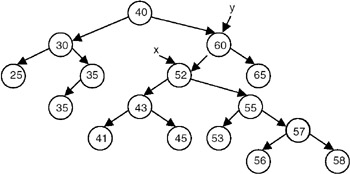Figure 21.17: A binary tree before deletion of a node pointed to by x.

To delete a node printed to by x, we start by letting y be a pointer to the node that is the root of the node pointed to by x. We store the pointer to the left child of the node pointed to by x in a temporary pointer temp. We then make the left child of the node pointed to by y the left child of the node pointed to by x. We then traverse the tree with the root as the node pointed to by temp to get its right leaf, and make the right child of this right leaf the right child of the node pointed to by x, as shown in Figure 21.18.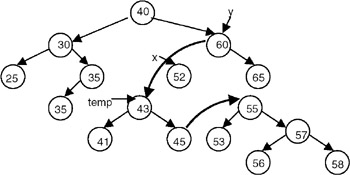Figure 21.18: A binary tree after deletion of a node pointed to by x.

Another method is to store the pointer to the right child of the node pointed to by x in a temporary pointer temp. We then make the left child of the node pointed by y to be the right child of the node pointed to by x. We then traverse the tree with the root as the node pointed to by temp to get its left leaf, and make the left child of this left leaf the left child of the node pointed to by x, as shown in Figure 21.19.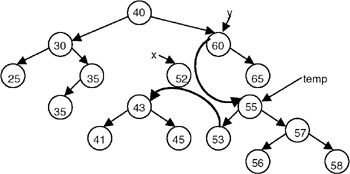Figure 21.19: A binary tree after deletion of a node pointed to by x.

Deletion of a Node with One Child

Consider the binary search tree shown in Figure 21.20.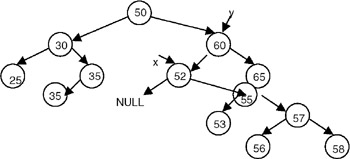Figure 21.20: A binary tree before deletion of a node pointed to by x.

If we want to delete a node pointed to by x, we can do that by letting y be a pointer to the node that is the root of the node pointed to by x. Make the left child of the node pointed to by y the right child of the node pointed to by x, and dispose of the node pointed to by x, as shown in Figure 21.21.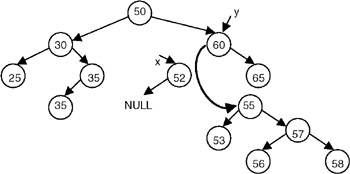Figure 21.21: A binary tree after deletion of a node pointed to by x.

Deletion of a Node with No Child

Consider the binary search tree shown in Figure 21.22.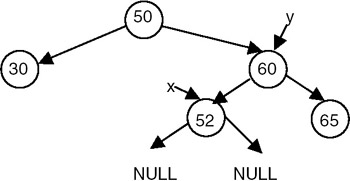Figure 21.22: A binary tree before deletion of a node pointed to by x.

Set the left child of the node pointed to by y to NULL, and dispose of the node pointed to by x, as shown in Figure 21.23.Figure 21.23: A binary tree after deletion of a node pointed to by x.

Program

A complete C program to delete a node, where the data value of the node to be deleted is known, is as follows:

```#include
#include
struct tnode
{
int data;
struct tnode *lchild, *rchild;
};
/* A function to get a pointer to the node whose data value is given
as well as the pointer to its root */
struct tnode *getptr(struct tnode *p, int key, struct tnode **y)
{
struct tnode *temp;
if( p == NULL)
return(NULL);
temp = p;
*y = NULL;
while( temp != NULL)
{
if(temp->data == key)
return(temp);
else
{
*y = temp; /*store this pointer as root */
if(temp->data > key)
temp = temp->lchild;
else
temp = temp->rchild;
}
}
return(NULL);
}

/* A function to delete the node whose data value is given */
struct tnode *delete(struct tnode *p,int val)
{
struct tnode *x, *y, *temp;
x = getptr(p,val,&y);
if( x == NULL)
{
printf("The node does not exists
");
return(p);
}
else
{
/* this code is for deleting root node*/
if( x == p)
{
temp = x->lchild;
y = x->rchild;
p = temp;
while(temp->rchild != NULL)
temp = temp->rchild;
temp->rchild=y;
free(x);
return(p);
}
/* this code is for deleting node having both children */
if( x->lchild != NULL && x->rchild != NULL)
{

if(y->lchild == x)
{
temp = x->lchild;
y->lchild = x->lchild;
while(temp->rchild != NULL)
temp = temp->rchild;
temp->rchild=x->rchild;
x->lchild=NULL;
x->rchild=NULL;
}
else
{
temp = x->rchild;
y->rchild = x->rchild;
while(temp->lchild != NULL)
temp = temp->lchild;
temp->lchild=x->lchild;
x->lchild=NULL;
x->rchild=NULL;
}

free(x);
return(p);
}
/* this code is for deleting a node with on child*/
if(x->lchild == NULL && x->rchild !== NULL)
{
if(y->lchild == x)
y->lchild = x->rchild;
else
y->rchild = x->rchild;
x->rchild; = NULL;
free(x);
return(p);
}
if( x->lchild != NULL && x->rchild == NULL)
{
if(y->lchild == x)
y->lchild = x->lchild ;
else
y->rchild = x->lchild;
x->lchild = NULL;
free(x);
return(p);
}
/* this code is for deleting a node with no child*/
if(x->lchild == NULL && x->rchild == NULL)
{
if(y->lchild == x)
y->lchild = NULL ;
else
y->rchild = NULL;
free(x);
return(p);
}
}
}
/*an iterative function to print the binary tree in inorder*/
void inorder1(struct tnode *p)
{
struct tnode *stack;
int top;
top = −1;
if(p != NULL)
{
top++;
stack[top] = p;
p = p->lchild;
while(top >= 0)
{
while ( p!= NULL)/* push the left child onto stack*/
{
top++;
stack[top] =p;
p = p->lchild;
}
p = stack[top];
top-;
printf("%d	",p->data);
p = p->rchild;
if ( p != NULL) /* push right child*/
{
top++;
stack[top] = p;
p = p->lchild;
}
}
}
}
/* A function to insert a new node in binary search tree to get a tree created*/
struct tnode *insert(struct tnode *p,int val)
{
struct tnode *temp1,*temp2;
if(p == NULL)
{
p = (struct tnode *) malloc(sizeof(struct tnode)); /* insert the new node as root node*/
if(p == NULL)
{
printf("Cannot allocate
");
exit(0);
}
p->data = val;
p->lchild=p->rchild=NULL;
}
else
{
temp1 = p;
/* traverse the tree to get a pointer to that node whose child will be the newly created node*/
while(temp1 != NULL)
{
temp2 = temp1;
if( temp1 ->data > val)
temp1 = temp1->lchild;
else
temp1 = temp1->rchild;
}
if( temp2->data > val)
{
temp2->lchild = (struct tnode*)malloc(sizeof(struct tnode));/ *inserts the newly created node
as left child*/
temp2 = temp2->lchild;
if(temp2 == NULL)
{
printf("Cannot allocate
");
exit(0);
}
temp2->data = val;
temp2->lchild=temp2->rchild = NULL;
}
else
{
temp2->rchild = (struct tnode*)malloc(sizeof(struct tnode));/ *inserts the newly created node
as left child*/
temp2 = temp2->rchild;
if(temp2 == NULL)
{
printf("Cannot allocate
");
exit(0);
}
temp2->data = val;
temp2->lchild=temp2->rchild = NULL;
}
}
return(p);
}

void main()
{
struct tnode *root = NULL;
int n,x;
printf("Enter the number of nodes in the tree
");
scanf("%d",&n);
while( n - > 0)
{
printf("Enter the data value
");
scanf("%d",&x);
root = insert(root,x);
}
printf("The created tree is :
");
inorder1(root);
printf("
Enter the value of the node to be deleted
");
scanf("%d",&n);
root=delete(root,n);
printf("The tree after deletion is
");
inorder1(root);
}
```

Explanation

This program first creates a binary tree with a specified number of nodes with their respective data values. It then takes the data value of the node to be deleted, obtains a pointer to the node containing that data value, and obtains another pointer to the root of the node to be deleted. Depending on whether the node to be deleted is a root node, a node with two children a node with only one child, or a node with no children, it carries out the manipulations as discussed in the section on deleting a node. After deleting the specified node, it returns the pointer to the root of the tree.

• Input: 1. The number of nodes that the tree to be created should have
2. The data values of each node in the tree to be created
3. The data value in the node to be deleted
• Output: 1. The data values of the nodes in the tree in inorder before deletion
2. The data values of the nodes in the tree in inorder after deletion

Example

• Input: 1. The number of nodes taht the created tree should have = 5
2. The data values of the nodes in the tree to be created are: 10, 20, 5, 9, 8
3. The data value in the node to be deleted = 9
• Output: 1.5 8 9 10 20
2 5 8 10 20

### Applications of Binary Search Trees

One of the applications of a binary search tree is the implementation of a dynamic dictionary. This application is appropriate because a dictionary is an ordered list that is required to be searched frequently, and is also required to be updated (insertion and deletion mode) frequently. So it can be implemented by making the entries in a dictionary into the nodes of a binary search tree. A more efficient implementation of a dynamic dictionary involves considering a key to be a sequence of characters, and instead of searching by comparison of entire keys, we use these characters to determine a multi-way branch at each step. This will allow us to make a 26-way branch according to the first letter, followed by another branch according to the second letter and so on.

### General Comments on Binary Trees

1. Trees are used to organize a collection of data items into a hierarchical structure.
2. A tree is a collection of elements called nodes, one of which is distinguished as the root, along with a relation that places a hierarchical structure on the node.
3. The degree of a node of a tree is the number of descendants that node has.
4. A leaf node of a tree is a node with a degree equal to 0.
5. The degree of a tree is the maximum of the degree of the nodes of the tree.
6. The level of the root node is 1, and as we descend the tree, we increment the level of each node by 1.
7. Depth of a tree is the maximum value of the level for the nodes in the tree.
8. A binary tree is a special case of tree, in which no node can have degree greater than 2.
9. The maximum number of nodes at level i in a binary tree is 2i−1.
10. The maximum number of nodes in a binary tree of depth k is 2k−1.
11. A complete binary tree of depth k is a tree with n nodes in which these n nodes can be numbered sequentially from 1 to n.
12. If a binary tree is a complete binary tree, it can be represented by an array capable of holding n elements where n is the number of nodes in a complete binary tree.
13. Inorder, preorder, and postorder are the three commonly used traversals that are used to traverse a binary tree.
14. In inorder traversal, we start with the root node, visit the left subtree first, then process the data of the root node, followed by that of the right subtree.
15. In preorder traversal, we start with the root node. First we process the data of the root node, then visit the left subtree, then the right subtree.
16. In postorder traversal, we start with the root node, visit the left subtree first, then visit the right subtree, and then process the data of the root node.
17. To construct a unique binary tree, we require two orders of traversal, in which one has to be inorder; the other could be preorder or postorder.
18. A binary search tree is an important search structure that is dynamic and allows a search by using O(log2n) steps.

Exercises

1. Write a C program to count the number of non-leaf nodes of a binary tree.
2. Write a C program to delete all the leaf nodes of a binary tree.
3. How many binary trees are possible with three nodes?
4. Write a C program to construct a binary tree with inorder and preorder traversals. Test it for the following inorder and preorder traversals:

• Inorder: 5, 1, 3, 11, 6, 8, 4, 2, 7
• Preorder: 6, 1, 5, 11, 3, 4, 8, 7, 2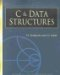C & Data Structures (Charles River Media Computer Engineering)
ISBN: 1584503386
EAN: 2147483647
Year: 2006
Pages: 232